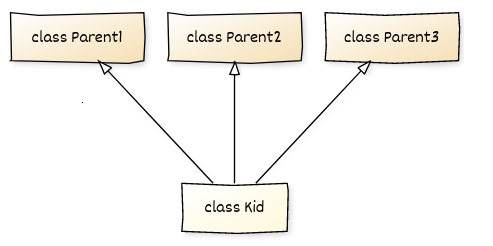# Python Multiple Inheritance

Multiple inheritances simply state that a child class (also known as a derived class) can inherit (use) the properties of the parent class ( also known as a base class). It helps to achieve the reusability of code.

Let us understand by an example.Explanation: In the above example there are 3 parent classes and a child class present. Now the arrows from the child class to parent1,parent2, and parent3 simply indicate that the child class has inherited the properties of all the 3 parent classes and can access their code when required.

Now let’s know some advantages of using inheritance

1. It allows the code to be reused as many times as needed
2. The base class once defined and once it is compiled, need not be reworked
3. Saves time and effort as the main code need not be written again

Example1: Now let us code a simple program where class A and Class B are parent class C is a child class.

Solution:

Code showing multiple inheritances where class C will print the line present in parent class A and B.

Code:

## Python Code

``````class A:
def a(self):
print('hello i am parent class 1')

class B:
def b(self):
print('hello i am parent class 2')

class C(A, B):
def c(self):
print('hello i am child class of parent class 1 and parent class 2')

c1 = C()
c1.a()
c1.b()
c1.c()``````

Output:

hello i am parent class 1
hello i am parent class 2
hello i am child class of parent class 1 and parent class 2

Example2: Now let’s try to code a program where the user will enter the required data and all the entered data will be displayed at the end.

Question: We have two parent classes Per_info and Edu_info and one child class named Student and implementing multiple inheritances.

Code:

## Python Code

``````class Per_info:
def __init__(self):
self.__enrollment=0
self.__name=""
self.__bloodgroup=""

def setPer_info(self):
self.__enrollment=int(input("Enter Enrollment no. "))
self.__name = input("Enter Name: ")

def showPer_info(self):
print("Enrollment no. ",self.__enrollment)
print("Name: ",self.__name)
print("bloodgroup: ",self.__bloodgroup)

class Edu_info:
def __init__(self):
self.__branch=""
def setEdu_info(self):
self.__branch=input("Enter branch: ")
def showEdu_info(self):
print("branch: ",self.__branch)

class Student(Per_info,Edu_info):
def __init__(self):
self.__city = ""
self.__contact = ""
def setStudent(self):
self.setPer_info()
self.__city = input("Enter your city: ")
self.__contact = input("Enter Contact: ")
self.setEdu_info()

def showStudent(self):
self.showPer_info()
print("city: ",self.__city)
print("Contact: ",self.__contact)
self.showEdu_info()

def main():
s=Student()
s.setStudent()
s.showStudent()
if __name__=="__main__":main()``````

Output: